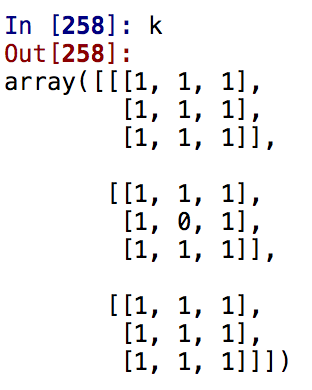# Extracting Voxels in the Legion Areas of the Brain

How to divide voxels into 3 groups?

1. I will have a NIFTY file (3D image of the patient with lesion in the brain). The voxels in the lesion region is labeled with the letter ‘L’. So, I need to find all voxels (only in lesion places) which surrounded from all sides with the voxels labeled as ‘L’ as well. So, if the voxel’s all neighbors (26 voxels) has the same label ‘L’, then I need show it.
2. Show only the voxels which are isolated. So, if the voxel’s all neighbors (26 voxels) are not labeled with the letter ‘L’, this should be isolated voxel, this is a different script, so the output is the different.
3. Last one is finding voxels with partial neighbors with the letter ‘L’. What I mean is that, here, even if a voxel is not fully covered by other voxels with the letter ‘L’ should be fine. A voxel might be covered only beneath or from the left side, and so on.

Please, could you help me and advise using which projects such as nipype, dipy, mindboggle, nibabel, Scitran SDM, Nipy, Nitime, popeye, Nilearn, PyMVPA, MNE, niwidgets I may achieve those results.

Best regards, Bissenbay!

Hi Bissenbay,

It’s not really clear what your inputs and outputs are, here, but I’m going to assume that you always have/want NIfTI-1 files. So let’s say you have:

1. `T1w.nii.gz` - your structural image
2. `lesion_mask.nii.gz` - an image that is 1 where the lesion is and 0 elsewhere

And what you want is:

1. `eroded_mask.nii.gz` - A mask where a voxel is 1 iff it and all of its neighbors are 1 in `lesion_mask.nii.gz`
2. `isolated_voxels.nii.gz` - A mask where a voxel is 1 iff it and none of its neighbors are 1 in `lesion_mask.nii.gz`
3. `cleaned_mask.nii.gz`- The `xor` of `lesion_mask.nii.gz` and `isolated_voxels.nii.gz`

So you should be able to do this all with nibabel and numpy.

``````import nibabel as nb
import numpy as np
``````

Now from what I can tell, there’s no actual need to work with the structural image, so we’ll work with the `lesion_mask.nii.gz`:

``````orig_mask = nb.load('lesion_mask.nii.gz')
``````

Now, you said that you had `'L'` instead of 1 to indicate the mask, so I’m not really sure what data you’re actually working with, but you can get to a binary mask from a `numpy` array of strings like so:

``````data = np.array(data == 'L', dtype=np.uint8)
``````

In any event, your data ought to be binary:

``````assert np.array_equal(np.unique(data), [0, 1])
``````

From here, you can now use all of the tools of `scipy.ndimage` on your array to perform erosion, and identify isolated voxels. One function you might find useful is `scipy.ndimage.interpolation.shift`, if you need to manually make copies of your array that are shifted.

Suppose that we’ve now created new data arrays that fit your requirements. You’ll want to create new NIfTI images out of those data arrays. To keep things in the same space, we’ll keep the original affine and header:

``````eroded_img = nb.Nifti1Image(eroded_data, affine=orig_mask.affine,
``````

Because we’re using binary images, to be safe, let’s set the on-disk data type to a single byte:

``````eroded_img.set_data_dtype(np.uint8)
``````

Then we can save the image:

``````eroded_img.to_filename('eroded_mask.nii.gz')
``````

I’ve made some assumptions, such as you’re using NIfTI-1 images… you can do basically the same with NIfTI-2, just making the obvious change to `Nifti2Image`. (More generally, you can let nibabel do the work for you by invoking `orig_mask.__class__(data, array, header)`, but that’s mild Python magic, so if it’s not clear what’s going on there, you can ignore this comment.)

Hopefully this can get you started. There are definitely a lot of holes to fill in, so feel free to ask for further guidance.

1 Like

Hi Chris,

Thank you for the meticulous explanation. Because I am a novice in this area that was hard to me to explain it coherently. However, you got my point.

Here is another question:
First, I am loading the NifTI image using nibabel and further will be working with 3d array (ndarray in numpy) where it stored 0 or 1 (meaning lesion) and x,y,z coordinates are the indices of the array.
Now, I need to find out the number of neighbor voxels with lesion of each lesion voxel. Of course, I understand that I can run a 3 nested for loop and according check their 26 neighbor voxels and if they contain 1, then count. However, I would really want to use the advantages numpy provides us such as broadcasting.
Let’s assume the variable name of the 3d array is lesion_data:
So, I can this sort of piece of code and have 10 added in all places where voxel is lesion.
lesion_data[lesion_data == 1.] = lesion_data[lesion_data == 1.] + 10

What do you think is there any easy way of having the similar approach to calculate the number of lesion neighbor voxels instead of running nested loop.

Any suggestions are welcome!

Thanks one more time.Your effort is really appreciated!

Hi Chris,

I found some solution.
Could you please if that is correct:

k = np.ones((3,3,3), dtype=int)
k[1,1,1] = 0lesion_neighbors = ndimage.convolve(lesion_data, k, mode=‘constant’, cval=0.0)

Hi Chris,
The solution I came up is doing the calculation on the whole array. However, I would like only do for the voxels which are 1. Is there any way of doing so?